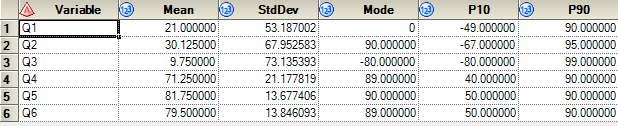## Proc Means and Proc Univariate Differences

Hello,

I wonder, do proc means procedure's and proc univariate procedure's functions calculate the same values. Firstly i wrote the procedure with using proc means now i want to try it with proc univariate but i got error i think their syntax is different. How can i write proc means code  as proc univariate

``````proc means data=WORK.sample STACKODS
FW=12
PRINTALLTYPES
CHARTYPE
QMETHOD=OS
VARDEF=DF
MEAN
STD
MODE
P10
P90 ;
var Q:;
ods output summary=stacked;
run;``````
``````proc univariate data=WORK.sample STACKODS
FW=12
PRINTALLTYPES
CHARTYPE
QMETHOD=OS
VARDEF=DF
MEAN
STD
MODE
P10
P90 ;
var Q:;
ods output summary=stacked;
run;``````

Thank you.

1 ACCEPTED SOLUTION

Accepted Solutions

## Re: Proc Means and Proc Univariate Differences

You have an extra close parenthesis. Remove the right parenthesis after your list of variables to keep. Dataset options are enclosed with () following the dataset name.   They are space delimited and in the form of option=value.  Some like the RENAME= option require their own set of () since the values contain equal signs. Formatting the code can make it easier to insure that they are properly nested.

``````mydsn
(keep= x y x
rename=
(x=a
y=b
)
obs=10
firstobs=3
)``````

13 REPLIES 13

## Re: Proc Means and Proc Univariate Differences

Please look in the online help as there are significant differences in syntax. As a hint see what you get with

``````proc univariate data=WORK.sample ;
var Q:;

run;``````

Printed appearance of decimal values may appear different due to different default widths of values displayed.

## Re: Proc Means and Proc Univariate Differences

Yes of course tried the statement that you wrote but ı try to get same output like below.Thank you.

## Re: Proc Means and Proc Univariate Differences

I don't think you easily can. Proc Univariate does not support the same output format in general. What do you want from proc univariate that you can't get in proc means?

## Re: Proc Means and Proc Univariate Differences

Okay then. Some of my friends said to me,univariate can generate different values but he is not sure, as you said there is no differences. I wanted to be sure. Thanks. Well,  how can we generate same output with univariate ?

Thank you.

## Re: Proc Means and Proc Univariate Differences

You can use the OUTPUT statement to generate the dataset and then you'd have to transpose it using proc transpose.

Or you can capture several of the ODS tables, merge them and/or transpose.

The documentation is fairly clear on how to get output from PROC UNIVARIATE, unlike proc means you do need to explicitly list variable names as well.

Proc univariate will generate same values for the same statistics, but it will also generate specific percentiles points which is when I generally use it over proc means.

https://support.sas.com/documentation/cdl/en/procstat/63104/HTML/default/viewer.htm#procstat_univari...Ksharp
Super User

## Re: Proc Means and Proc Univariate Differences

You can use the following code to get the same layout as proc means,but you can't customize it .

``````proc univariate data=sashelp.class outtable=want;
var weight;
run;``````

## Re: Proc Means and Proc Univariate Differences

Thank you , i'm so close to do it. Actually, at the first code i made it but i didn't bring the columns which i want it. Second code i got the columns but just for one Q. Have you got an any idea ?.

``````bdata sample;

length Q1 8 Q2 8 Q3 8 Q4 8 Q5 8 Q6 8;

infile datalines  missover dlm=',';

input Q1 Q2 Q3 Q4 Q5 Q6;

datalines;

80,90,70,90,80,70

90,95,99,40,50,90

0,90,99,89,87,89

77,88,0,45,78,89

0,0,0,58,90,89

-10,-10,-30,89,90,79

-20,-45,-80,89,90,50

-49,-67,-80,70,89,80

;

proc univariate data=work.sample outtable=sample;

var Q:;

run;

proc univariate data=work.sample2;

var _VAR_ _MEAN_  _STD_ _P10_ _P90_ _MODE_;

run;``````
``````proc univariate data=work.sample;

var Q:;

output out=univariateTable

mean=Mean

Mode=Mode

P10=P10

P90=P90

STDDEV=STDDEV;

run;``````Ksharp
Super User

## Re: Proc Means and Proc Univariate Differences

The table (want) generated by OUTTABLE= have already contain these statistic estimator.

You just need KEEP it .

``````data sample;
length Q1 8 Q2 8 Q3 8 Q4 8 Q5 8 Q6 8;
infile datalines  missover dlm=',';
input Q1 Q2 Q3 Q4 Q5 Q6;
datalines;
80,90,70,90,80,70
90,95,99,40,50,90
0,90,99,89,87,89
77,88,0,45,78,89
0,0,0,58,90,89
-10,-10,-30,89,90,79
-20,-45,-80,89,90,50
-49,-67,-80,70,89,80
;

proc univariate data=work.sample outtable=want(keep=_var_ _mean_ _std_ _mode_ _p10_ _p90_);
var Q:;
run;``````

## Re: Proc Means and Proc Univariate Differences

Yes I made it, is it possible to change columns name ? I tried but did not succeed.

Thank you.

``````proc univariate data=work.sample outtable=want(keep=_var_ _mean_ _std_ _mode_ _p10_ _p90_)
;
label _VAR_=Variable;
var Q:;
run;``````

## Re: Proc Means and Proc Univariate Differences

Yes. if you wanted to rename _VAR_ to VARIABLE you would  include it in the list of old=new names in the RENAME option on the output dataset name.

``````PROC UNIVARIATE data=sashelp.class
OUTTABLE=want
(
RENAME=(_VAR_ = VARIABLE
)
)
;
RUN;``````

## Re: Proc Means and Proc Univariate Differences

Yes it works tahnk you. But this time i couldn't  use drop or keep statement ?

``````PROC UNIVARIATE data=WORK.sample
OUTTABLE=want (keep=_var_)
(RENAME=(_VAR_ = VARIABLE));
var Q:;
RUN;``````

Thank you.

## Re: Proc Means and Proc Univariate Differences

You have an extra close parenthesis. Remove the right parenthesis after your list of variables to keep. Dataset options are enclosed with () following the dataset name.   They are space delimited and in the form of option=value.  Some like the RENAME= option require their own set of () since the values contain equal signs. Formatting the code can make it easier to insure that they are properly nested.

``````mydsn
(keep= x y x
rename=
(x=a
y=b
)
obs=10
firstobs=3
)``````

## Re: Proc Means and Proc Univariate Differences

Yes. That's it. Thank you 🙂

``````PROC UNIVARIATE data=WORK.sample OUTTABLE=want
(keep=_var_ _mean_ _std_ _mode_ RENAME=(
_VAR_=VARIABLE
_MEAN_=MEAN
_STD_=STDDEV
_MODE_=MODE
));
var Q:;
RUN;``````
Discussion stats
• 13 replies
• 5507 views
• 0 likes
• 5 in conversation